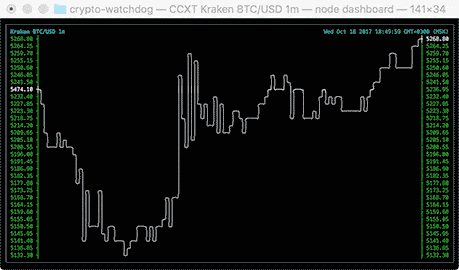asciichartpy Release 1.5.25

Nice-looking lightweight console ASCII line charts ╭┈╯ with no dependencies

Keywords
asciichart, ascii, chart, charting, charts, console, terminal, draw, box-drawing, box, drawing, stats, line, linear, plot, graph, graphics, command, commandline, bash, shell, sh, light, ansi, ascii-chart, browser, charting-library, console-log, javascript, js, line-charts, lines, node-js, nodejs, text-chart
MIT
Install
pip install asciichartpy==1.5.25

asciichart

Console ASCII line charts in pure Javascript (for NodeJS and browsers) with no dependencies. This code is absolutely free for any usage, you just do whatever the fuck you want.Usage

NodeJS

npm install asciichart
var asciichart = require ('asciichart')
var s0 = new Array (120)
for (var i = 0; i < s0.length; i++)
s0[i] = 15 * Math.sin (i * ((Math.PI * 4) / s0.length))
console.log (asciichart.plot (s0))

Browsers

<!DOCTYPE html>
<html>
<meta http-equiv="Content-Type" content="text/html; charset=utf-8">
<meta charset="UTF-8">
<title>asciichart</title>
<script src="asciichart.js"></script>
<script type="text/javascript">
var s0 = new Array (120)
for (var i = 0; i < s0.length; i++)
s0[i] = 15 * Math.sin (i * ((Math.PI * 4) / s0.length))
console.log (asciichart.plot (s0))
</script>
<body>
</body>
</html>

Options

The width of the chart will always equal the length of data series. The height and range are determined automatically.

var s0 = new Array (120)
for (var i = 0; i < s0.length; i++)
s0[i] = 15 * Math.sin (i * ((Math.PI * 4) / s0.length))
console.log (asciichart.plot (s0))The output can be configured by passing a second parameter to the plot (series, config) function. The following options are supported:

var config = {

offset:  3,          // axis offset from the left (min 2)
padding: '       ',  // padding string for label formatting (can be overrided)
height:  10,         // any height you want

// the label format function applies default padding
format:  function (x, i) { return (padding + x.toFixed (2)).slice (-padding.length) }
}

Scale To Desired Heightvar s = []
for (var i = 0; i < 120; i++)
s[i] = 15 * Math.cos (i * ((Math.PI * 8) / 120)) // values range from -15 to +15
console.log (asciichart.plot (s, { height: 6 }))     // this rescales the graph to ±3 linesAuto-range

var s2 = new Array (120)
s2 = Math.round (Math.random () * 15)
for (i = 1; i < s2.length; i++)
s2[i] = s2[i - 1] + Math.round (Math.random () * (Math.random () > 0.5 ? 2 : -2))
console.log (asciichart.plot (s2))A util by madnight for drawing Bitcoin/Ether/altcoin charts in command-line console: bitcoin-chart-cli.Ports

Special thx to all who helped port it to other languages, great stuff!

Future work (coming soon, hopefully)

• multi-line colored charts in console!
• levels and points on the graph!
• even better value formatting and auto-scaling!